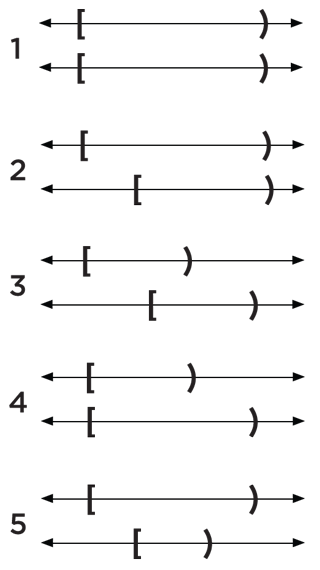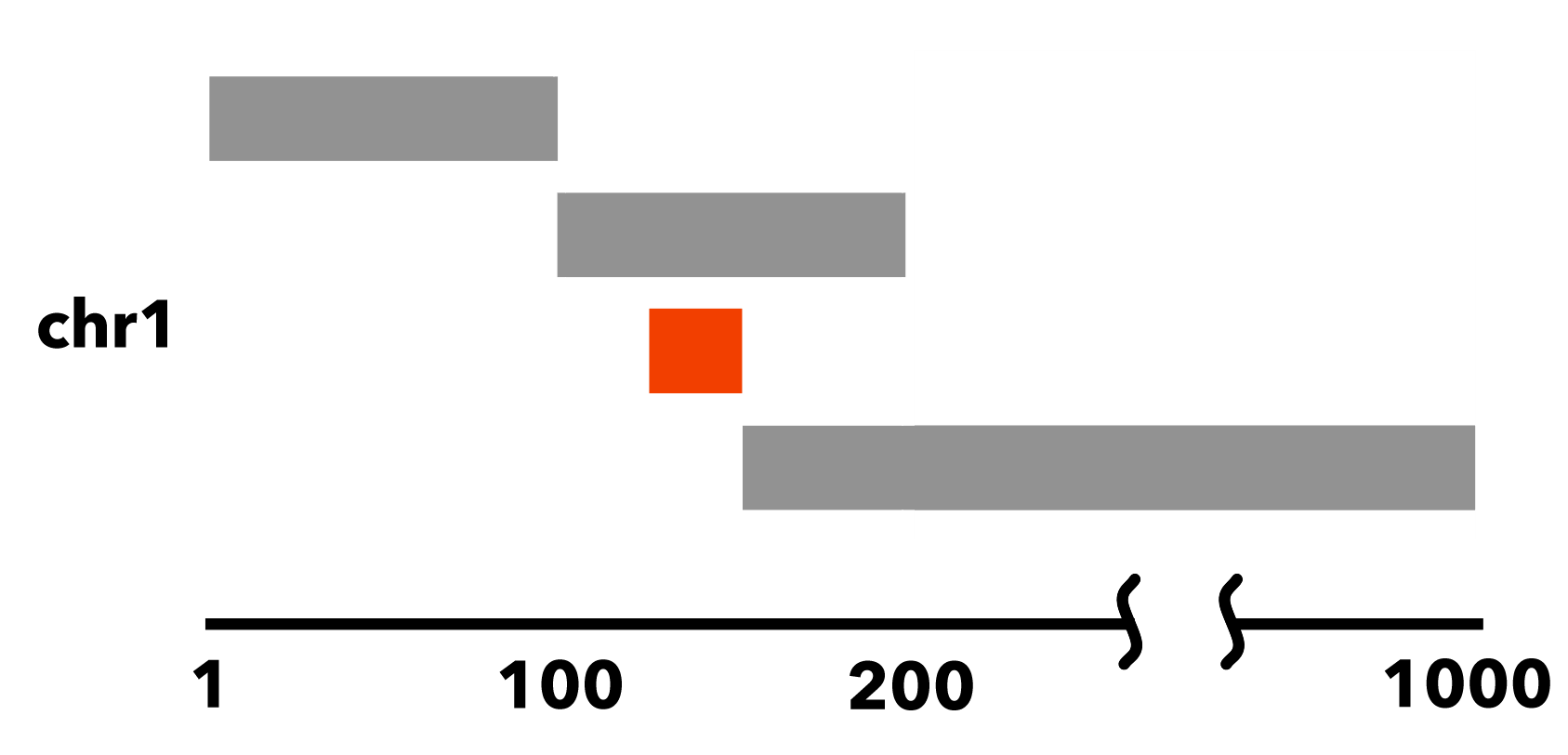# 6.1.4. Nested elements¶

This page describes nested BED elements, their impact on the performance of BEDOPS tools, and how we can identify them beforehand.

## 6.1.4.1. Definition¶

A nested element is defined as a BED element from a sorted BED file, where a genomic range is entirely enclosed by the previous element’s range.

Loosely speaking, consider the following five overlap cases for pairings of generic, half-open intervals:Of these five interval pairs, the fifth overlap pairing identifies a nested element, where the second interval is nested within the first.

More rigorously, we define two generic, half-open BED elements $$A$$ and $$B$$, both located on the same generic chromosome $$N$$, each with ranges $${[a_{start}, a_{stop})}$$ and $${[b_{start}, b_{stop})}$$, respectively.

These two elements $$A$$ and $$B$$ have the following relations:

1. $$a_{start} < a_{stop}$$
2. $$b_{start} < b_{stop}$$
3. $$a_{start} <= b_{start}$$
4. $$a_{stop} <= b_{stop}$$

Note

The third and fourth conditions place elements $$A$$ and $$B$$ into sort order, as applied by the sort-bed application.

If we further restrict these ranges: $$a_{start} < b_{start}$$ and $$b_{stop} < a_{stop}$$, then for the purposes of BEDOPS we call the element $$B$$ a nested element, one which is contained or nested within element $$A$$.

It can be useful to be able to identify nested elements in an input set. Here’s a method that uses awk:

#!/usr/bin/env awk -f
{
if (NR > 1) {
currentChr = $1 currentStart =$2
currentStop = $3 if ((previousStart < currentStart) && (previousStop > currentStop)) { print$0;
}
else {
previousChr = currentChr
previousStart = currentStart
previousStop = currentStop
}
}
else {
previousChr = $1 previousStart =$2
previousStop = \$3
}
}


If this script is given the name getNestedElements.awk and is made executable, one could filter a BED file via ./getNestedElements.awk foo.bed > nested.bed, or similar.

## 6.1.4.2. Example¶

A more concrete example of a sorted BED file which contains a nested element follows. Consider the following simple, sorted BED dataset:

chr1    1      100
chr1    100    200
chr1    125    150
chr1    150    1000


Here, the element chr1:125-150 is entirely nested within chr1:100-200:## 6.1.4.3. Why nested elements matter¶

BEDOPS bedmap and bedextract tools offer the --faster option to perform very fast retrieval of overlapping elements, so long as input datasets do not contain nested elements, as defined above.

To extract maximum performance out of the use of the BEDOPS toolkit, therefore, it is very useful to know if the input datasets contain such elements — if they do not, then we can apply this optimization.

Common datasets we work with do not contain nested elements: reads, peaks, footprints, and others. However, other datasets do, such as motif hits or paired-end sequencing reads.

How can we find out if our inputs have nested elements, before we start applying any operations?

The compression tool starch (v2.5 and greater) will look for these elements in an input BED file and store this condition as a flag in the output archive’s metadata. This value can be retrieved in constant time with unstarch and other tools which make use of the Starch C++ API.

Additionally, the --ec (error-correction) option in bedmap will also report if inputs contain nested elements. This option doubles execution time, but when used in conjunction with the --faster option, the speed gains are more than recovered.